# How to Make Super-Sharp, Vector SwordsRead Time: 10 mins

In this tutorial I'll show you how to draw a sword in Illustrator, using the Rectangle Tool, gradients, and other Illustrator tools. Actually the tutorial isn't very hard, let's see the final result that is our goal first!

### Final Image Preview

Below is the final image we will be working towards. Want access to the full Vector Source files and downloadable copies of every tutorial, including this one? Join Vector Plus for just 9\$ a month.

#### Tutorial Details

• Program: Adobe Illustrator CS4
• Difficulty: Intermediate
• Estimated Completion Time: 1.5 - 2 hours

Here is the illustration we'll be creating.

### Step 1

Open a new document, pick the Rectangle Tool (M), create a rectangle like the one shown below, and fill it with a linear gradient with colors from R=213, G=237, B=254 to R=142, G=158, B=171.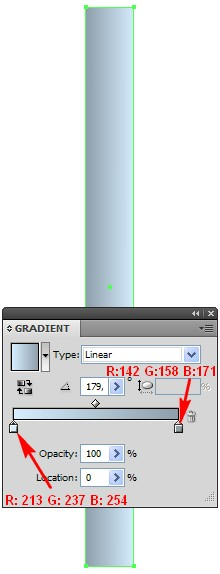### Step 2

Now pick the Add Anchor Point Tool (+) and add two points in the bottom part of the rectangle. Now use the Convert Anchor Point Tool (Shift + C) to edit the two key points that you added, as showed below. Select the bottom two points and go to Object > Path > Average (Alt + Command + J) and in the new open window turn on Both, and click OK.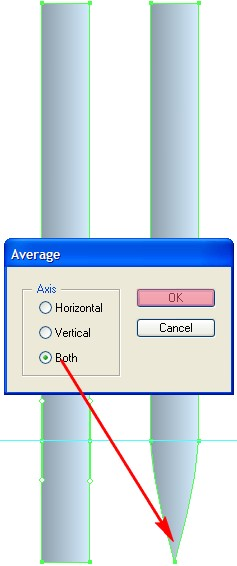### Step 3

Pick the Rectangle tool (M), create a rectangle in the bottom part of the first rectangle as shown.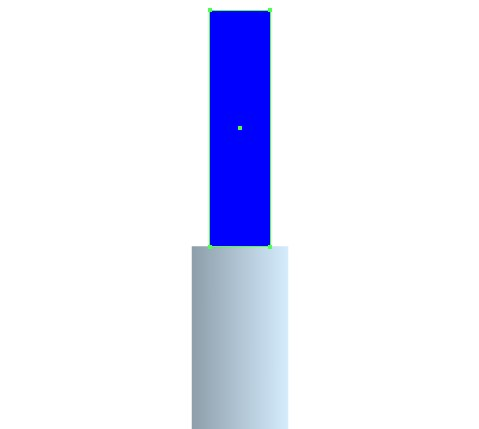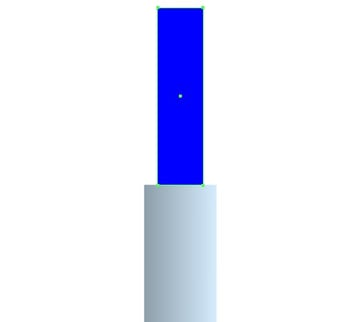### Step 4

With the rectangle you created earlier selected, pick the Add Anchor Point Tool (+), add two points in the positions as shown and edit them as indicated below. Fill them with the same gradient from Step 2, open the Pathfinder panel and click on Add to Shape Area.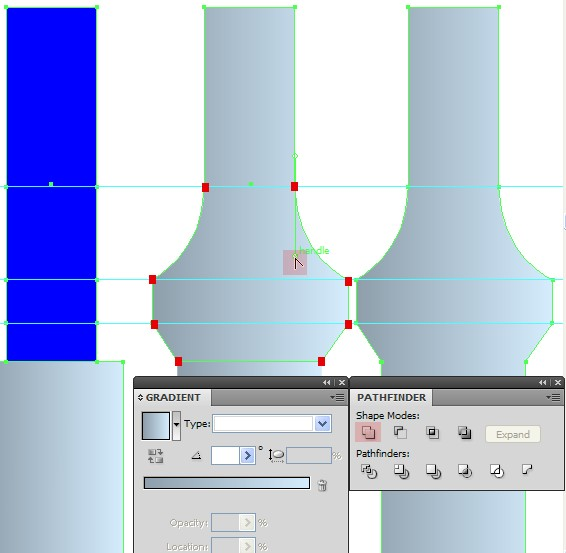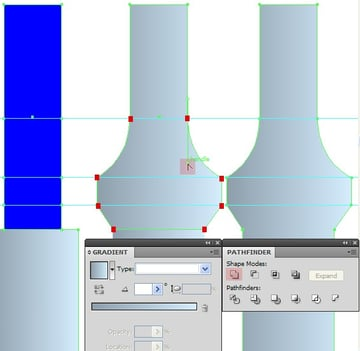### Step 5

Now copy the newly created shape from Step 4 using Command + C followed by Command + F to paste in front. Decrease the copy's size from the right and the left side. To do this, pick the Selection Tool (V), click on one of the side points, then press and hold the Alt key and slide it a little bit inwards.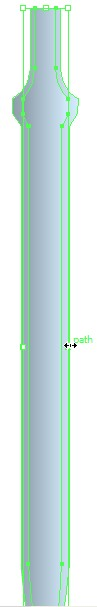### Step 6

With the shape whose size you just decreased still selected, pick the Direct Selection Tool (A) and delete the points on the right side. To do this, click on the point which has to be removed and press the Delete key. Then pick the Pen Tool (P), click on the top point in the top part in the middle, and then on the point at the bottom, and fill with a Linear Gradient.

Add three more Gradient sliders, for the first one from left to right select this color: R=30, G=73, B=123, for the second one R=54, G=102, B=162, for the third one R=0, G=97, B=148, for the fourth one R=91, G=127, B=173 and for the last fifth slider R=124, G=156, B=189.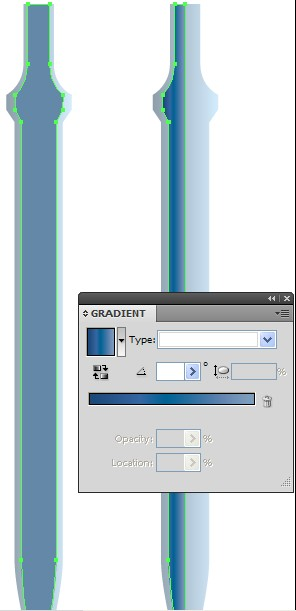### Step 7

Copy the shape you filled with a linear gradient and apply Command + C followed by Command + F, flip it horizontally, and then fill it with the color R=100, G=136, B=168.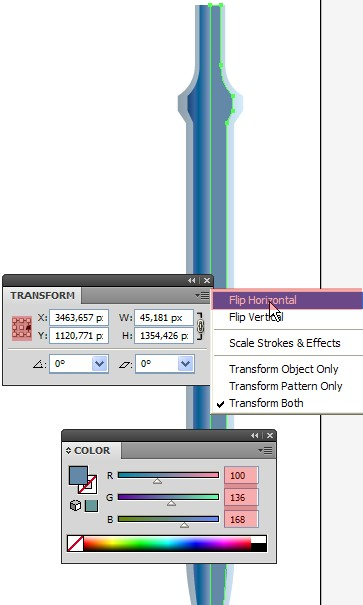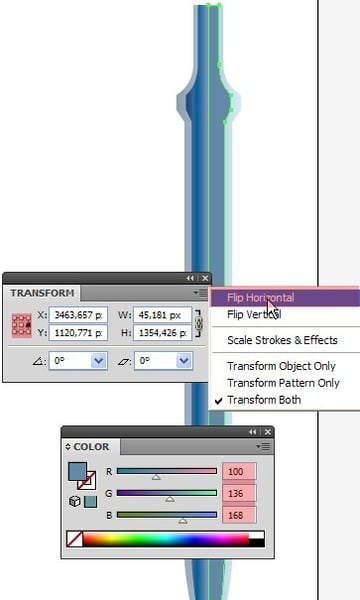### Step 8

Now copy the shape you filled with a linear gradient using Command + C followed by Command + F. Now turn off the fill color, delete the top-right point of the shape using the Direct Selection Tool (A), and apply a white Stroke with a weight of 1pt.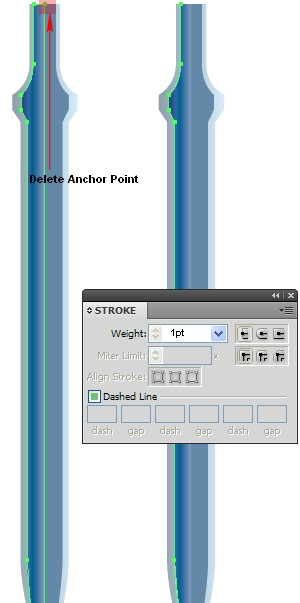### Step 9

Copy the shape you created in Step 4 using Command + C followed by Command + F. Remove the top points of the copy using the Direct Selection Tool (A), also remove the right point. Use the Add Anchor Point Tool (+) to add one point as shown below, then using the Direct Selection tool (A) edit the top key point as indicated. Now use the Pen Tool (P) to close the path like we did in the previous steps. Fill with color R=121, G=134, B=158 and reduce the Opacity to 70%.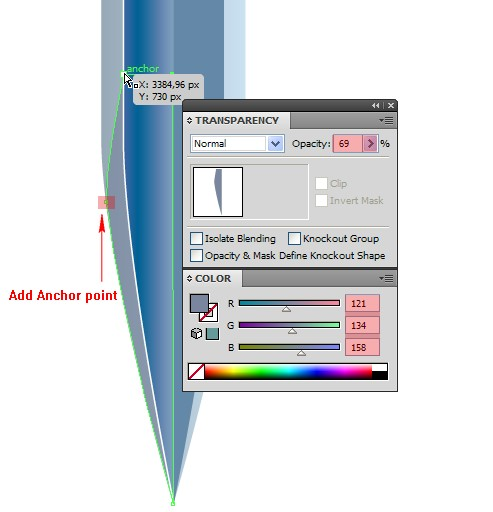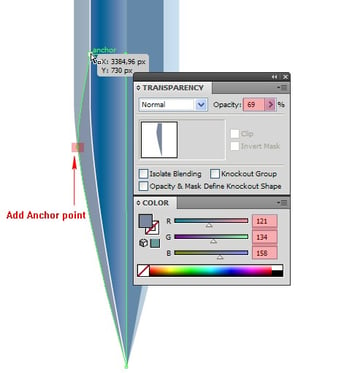### Step 10

Copy the last shape you created using Command + C followed by Command + F, flip it horizontally, change the Fill color to white and reduce the Opacity to 50%.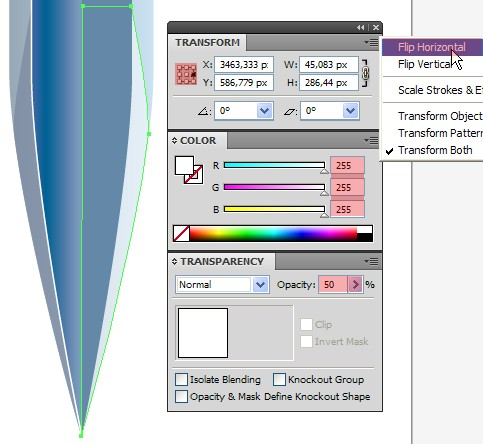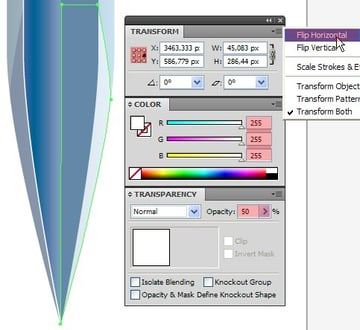### Step 11

Copy the shape from Step 4 once more using Command + C followed by Command + F. Now we need to move the shape on top of all the other shapes. With the copy still selected, go to Object > Arrange > Bring to Front.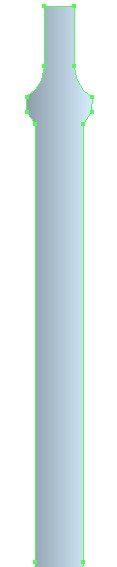### Step 12

As the shape is still selected, pick the Direct Selection Tool (A) and this time delete the bottom points of the shape and fill with a linear gradient as shown.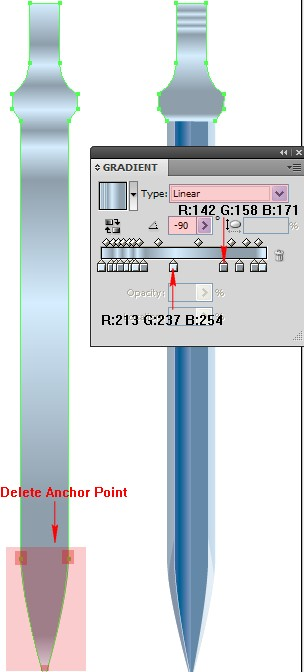### Step 13

Create a rectangle using the Rectangle Tool (M) on top of all the other shapes and position as shown, then fill it with a linear gradient. To do this, open the Gradient panel and select Type: Linear. You need 3 Stop color sliders all together. For the first one from left to right select color R=84, G=110, B=114, for the second one R=12, G=20, B=39, for the third one R=21, G=27, B=48 and reduce the Opacity to 75%.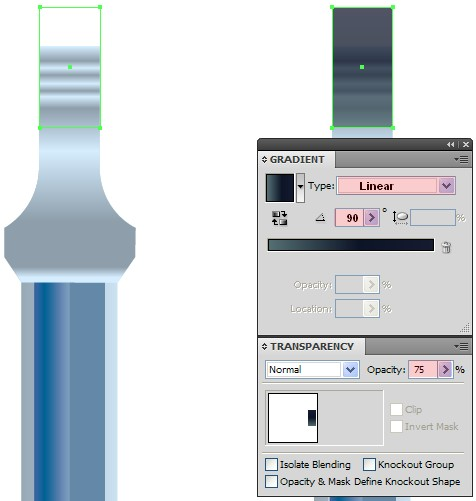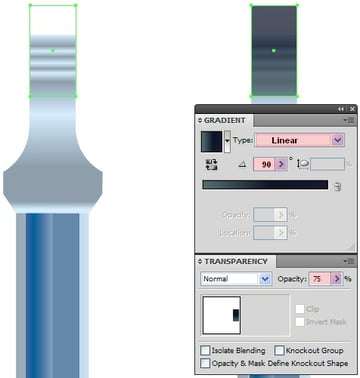### Step 14

Copy the shape from Step 4 using Command + C followed by Command + F. And now we need to move it on top of all the other shapes. To do this, go to Object > Arrange > Bring to Front.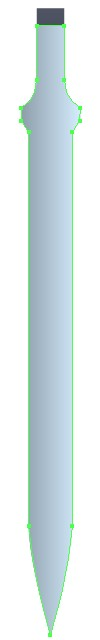### Step 15

With the shape we just copied still selected, pick the Selection Tool (V), decrease the size of the shape from the left and the right sides by clicking on one of the side points. Press and hold the Alt key and slide it a little bit inwards. Then pick the Direct Selection Tool (A), select the bottom 3 points of the shape and slide a few pixels upwards, and fill with a linear gradient.

To do this, open the Gradient panel and select Type: Linear. You need 3 Stop color sliders all together. For the first one from left to right apply color R=172, G=220, B=227, for the second one R=166, G=204, B=226 and for the third one R=160, G=199, B=231.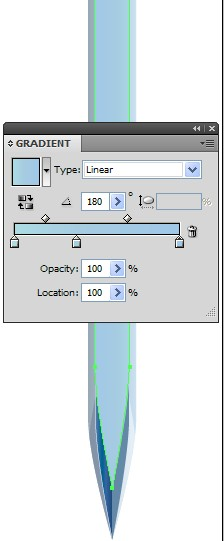### Step 16

Create another rectangle using the Rectangle Tool (M) as the one shown below, then fill it with Linear Gradient. To do this, open the Gradient panel and select Type: Linear. You need 3 Stop color sliders all together. For the first one from left to right select color R=12, G=20, B=39, for the second one R=84, G=94, B=114, for the third one R=21, G=27, B=48 and slide the top 2 points of the rectangle tool using the Direct Selection Tool (A) until they merge with the corners of the previous rectangle from Step 13.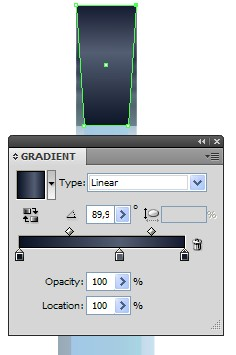### Step 17

Create shapes like the ones shown below using the Polygon Tool and the Rectangle Tool. Select both shapes, open the Pathfinder panel and click on Add to Shape Area. This way you'll get one whole shape.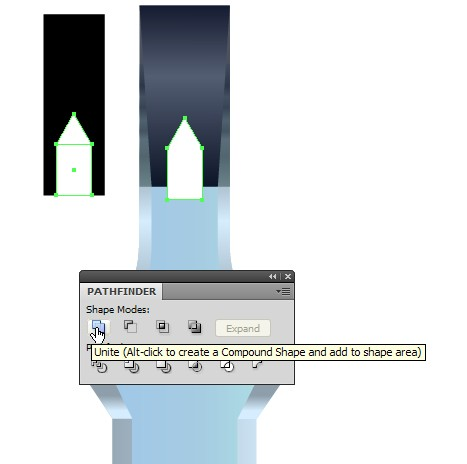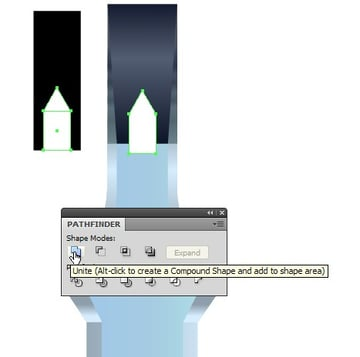### Step 18

Now select the newly created shape and the rectangle, open the Pathfinder panel and click on Minus Front.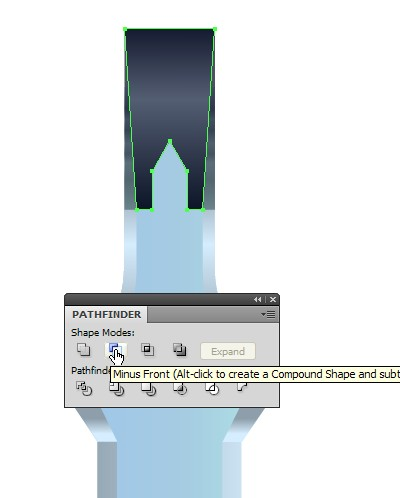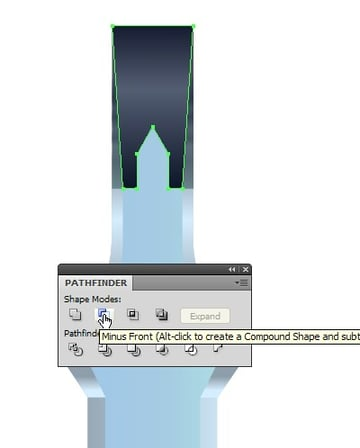### Step 19

Create a shape like the one shown below. Remove the top two points on the left side, and fill the shape with a linear gradient.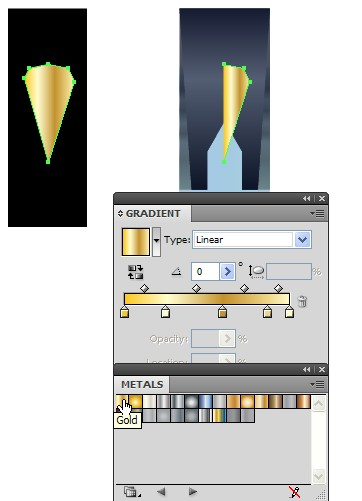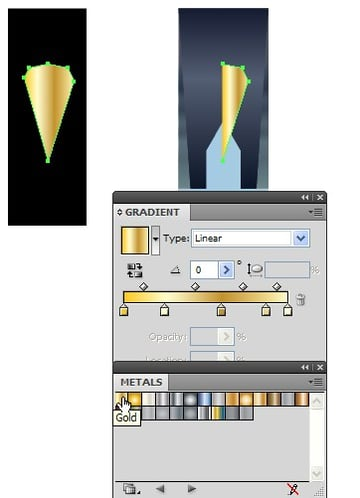### Step 20

Copy it using Command + C followed by Command + F, flip it horizontally and fill it with a little bit darker gradient.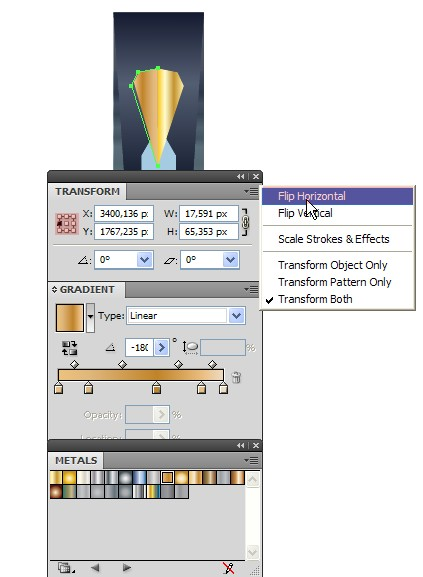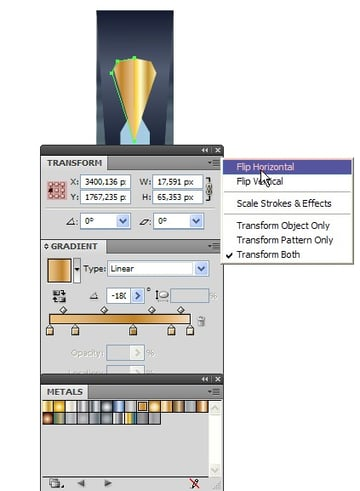### Step 21

Pick the Rectangle Tool (M) and create a rectangle. With it still selected, go to Effect > Stylize > Round Corner, set the Radius to 10px and click OK. Then go to Object > Expand Appearance and straight after that go to Object > Envelope Distort > Make With Warp. In the new open window select Style: Inflate, Bend: 50%, and click OK. Straight after that, go to Object > Expand Appearance and fill with color R=53, G=57, B=90.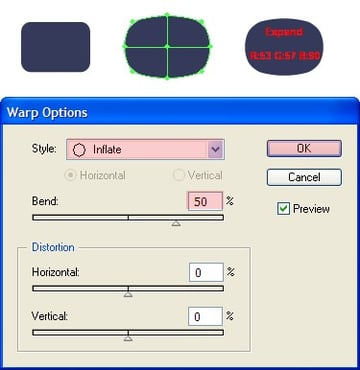### Step 22

Grab the Mesh Tool (U), click on a few places over the shape and select color for the points which are in the middle R=74, G=149, B=221. for the points on the left and right sides select color R=43, G=48, B=75, and position the shape as shown.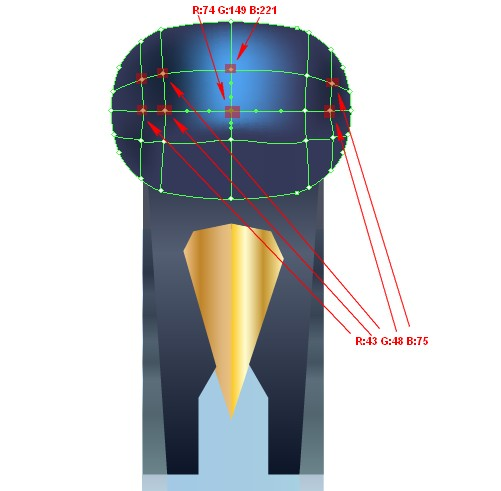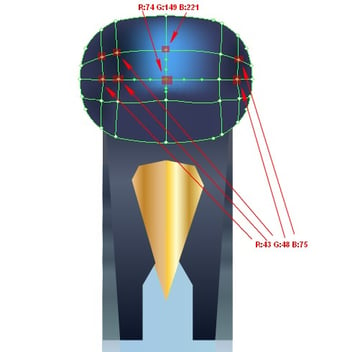### Step 23

Create another shape over the previous one with the same size and one rectangle, which will cover half of the previous shape. Open the Pathfinder panel and click on Minus Front.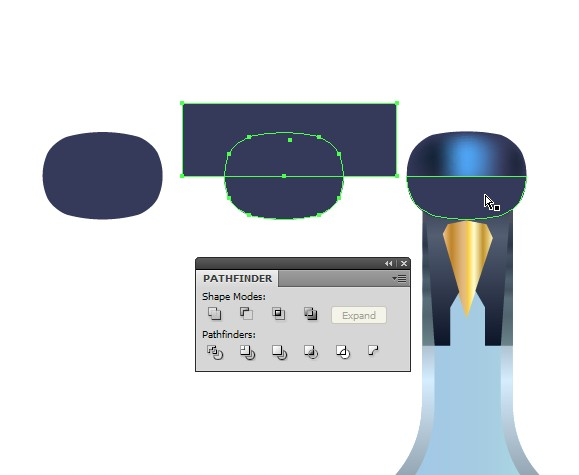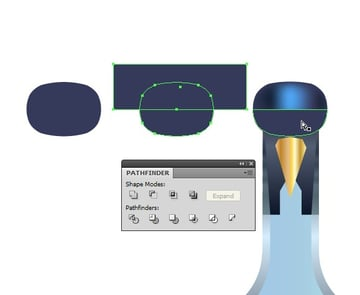### Step 24

Now copy the last created shape using Command + C followed by Command + F. Remove the bottom points of the shape using the Direct Selection Tool (A) so only the top two points remains. Go to Window > Brush Libraries > Artistic > Artistic Ink, select Tapered stroke and select a stroke color of white with Weight at 0,25pt.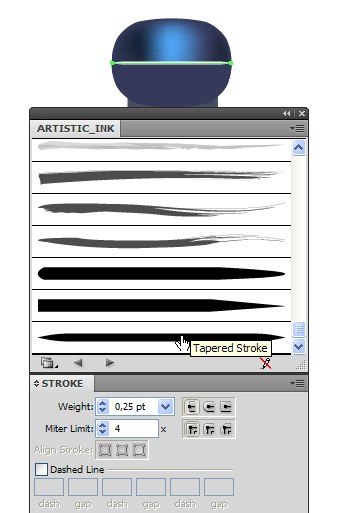### Step 25

Create a rectangle using the Rectangle Tool (M), which will represent the sword's handle and fill it with a Linear Gradient. To do this, open the Gradient panel (Command + F9) and select Type: Linear.

You need a 3 Stop color sliders all together. For the first one from left to right select a black color, for the second one R=84, G=78, B=114, for the third one R=12, G=20, B=39 and position it behind all the other shapes by going to Object > Arrange > Send to Back.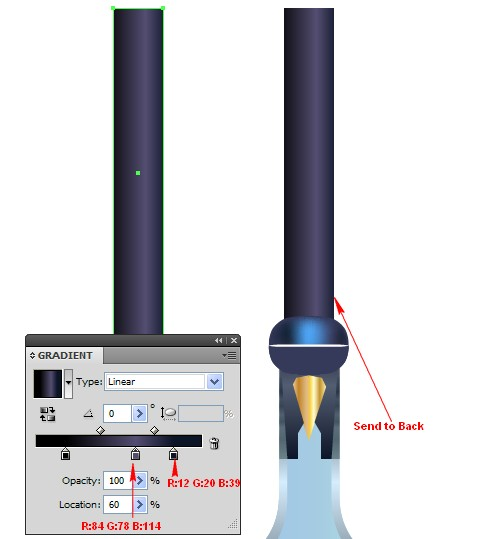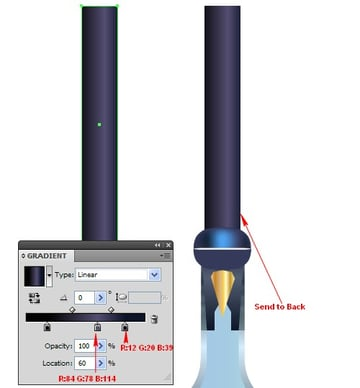### Step 26

Copy the shape that we applied colors with the Mesh Tool to using Command + C followed by Command + F to paste in front. Decrease the copy's size and position it on the handle's top.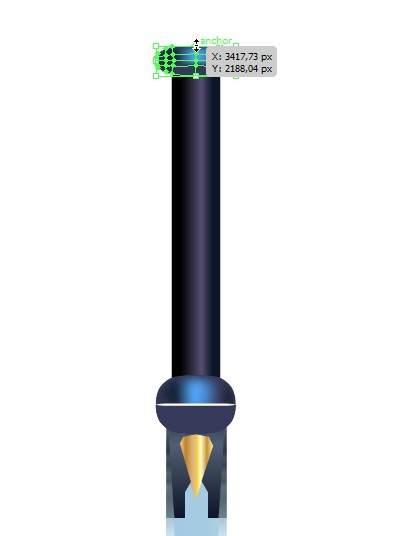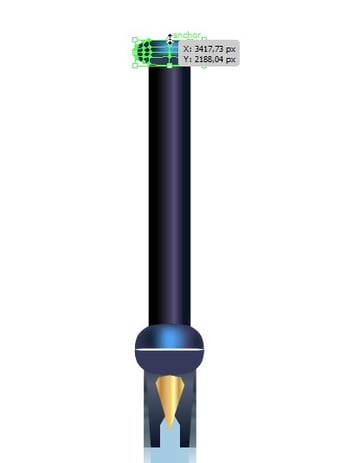### Step 27

Now we'll decorate the handle a bit by adding wings. To do them, create a rectangle and go to Object > Transform > Move, depending on how wide the rectangle you created is, you define the rectangle's width (the rectangle I've made is 25px wide) and select vertical is as much as your rectangle's width, and click Copy. Repeat these actions four more times using Command + D.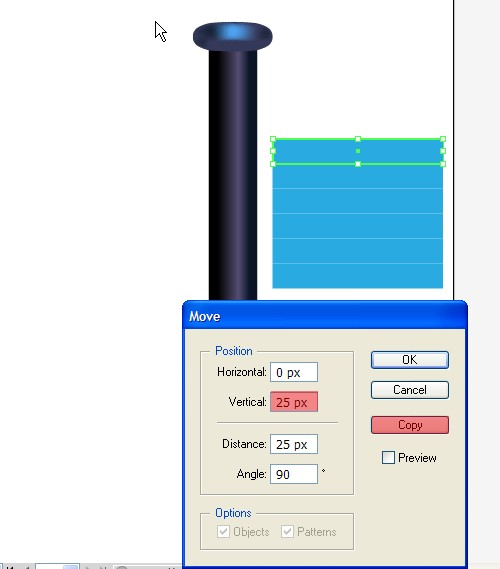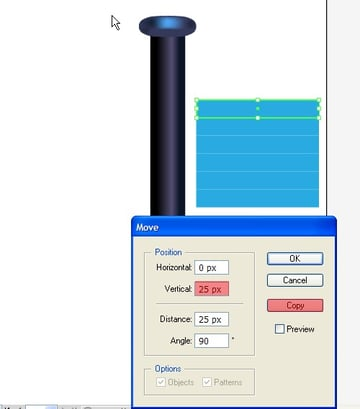### Step 28

Select the six rectangles you created earlier and go to Object > Envelope Distort > Make With Warp. In the new open window apply the following settings: Style of Arch, Bend of 50%, Distortion, Horizontal at 70%, Vertical at -25%, and click OK.

Straight after that go to Object > Expand, rotate the shapes to -90 degrees and fill them with a linear gradient. To do this, open the Gradient panel and select type: Linear. You need 2 Stop color sliders. For the first one apply color R=12, G=20, B=39, and for the second one R=96, G=91, B=140.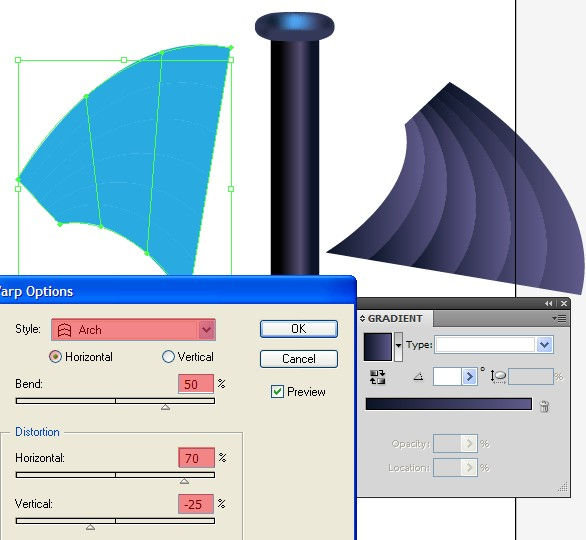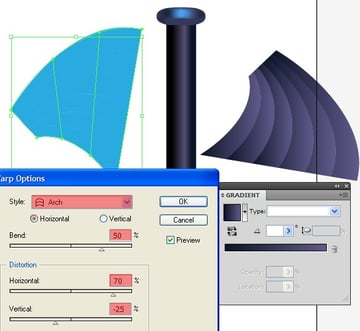### Step 29

Copy the wing using Command + C followed by Command + F, turn off the Fill color and apply a Stroke with a random color and a Weight of 1pt. Now go to Object > Path > Outline Stroke and fill with a linear gradient, by going to Window > Swatch Libraries > Gradients > Metal and select Gold.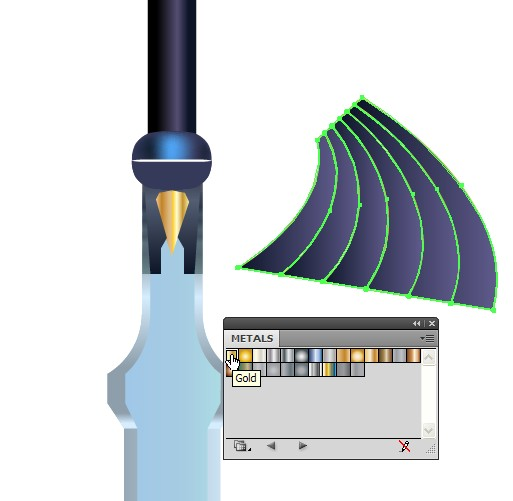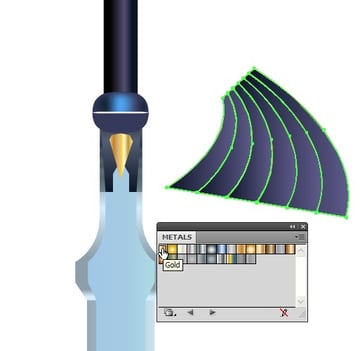### Step 30

Group all shapes of the wing by selecting them and going to Object > Group (Command + G). Copy the group with the wing using Command + C followed by Command + F to paste in front. Flip it horizontally, rotate it to 90 degrees, and decrease the copy's size a little bit. Now move it behind the original by applying Object > Arrange > Send to Backward.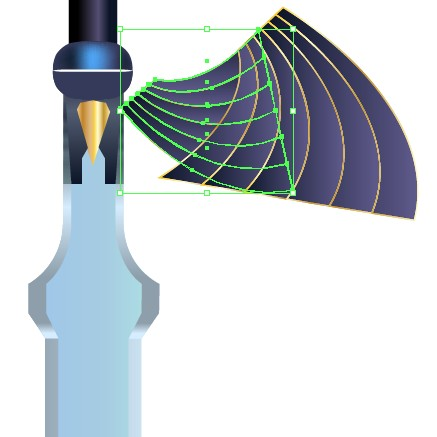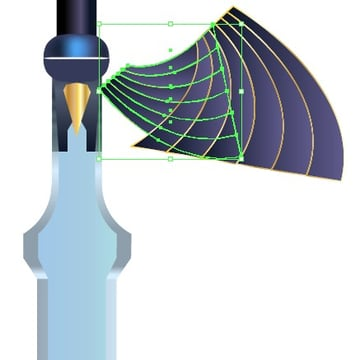### Step 31

Select the bigger and the smaller shape from the wing, group them by going to Object > Group and send them behind all other shapes by applying Object > Arrange > Send to Back. Copy the group with the wing using Command + C followed by Command + F and flip it horizontally.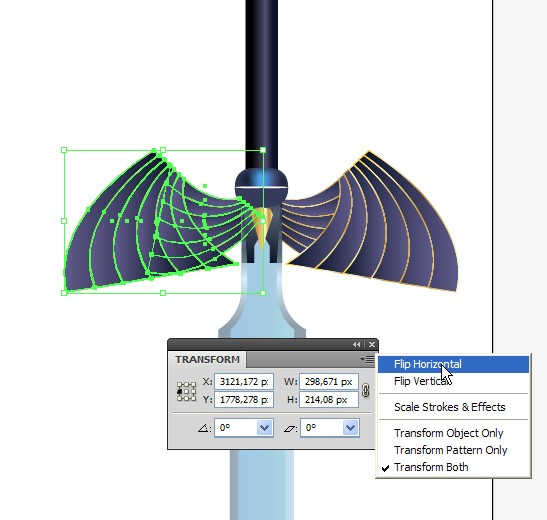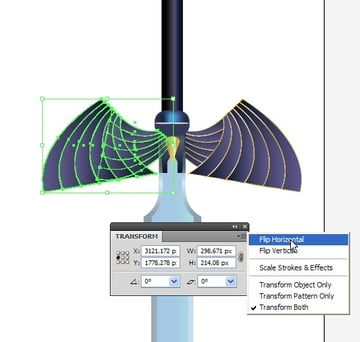In the end you can add a shadow and whatever else you decide.

### Final Image

This is what the final swords look like after adding a bit of blood and a simple background!

Subscribe to the Vectortuts+ RSS Feed to stay up to date with the latest vector tutorials and articles.